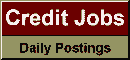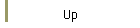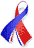DefaultRisk.com the web's biggest credit risk modeling resource.doi> search: A or B Export citation to:- HTML- Text (plain)- BibTeX- RIS- ReDIF

Inverse CIR and Semi-Affine Intensity-based Modeling on Credit Risk

by Chuang Yi of McMaster University, and
Tom Hurd of McMaster University

April 2008

Abstract: In this paper, we propose a semi-affine intensity-based model on credit risk. The default-free interest rate, r(t), is modeled as an inverse CIR (iCIR) process, which was firstly studied by Ahn & Gao (1999). The firm's short spread, h j (t), for firm j, is modeled as a linear combination of the default-free interest rate, the inverse of the interest rate and another idiosyncratic CIR factor. This model extends the Ahn & Gao (1999) one factor iCIR model on the interest rate to a multi-factor model on credit risk. This parsimonious model allows us to form rich correlation structures between the short spreads of different firms, while the non-negativity conditions for interest rates and short spreads are satisfied. The pricing formulae for default-free and defaultable bonds are non-affine in the common factor, but are affine in the idiosyncratic factor. These bond pricing formulae are obtained in explicit forms using the recent findings of stochastic integrals by Hurd & Kuznetsov (2006).

Keywords: iCIR, Intensity, Semi-Affine, Factor Models, Credit Spreads.

Books Referenced in this paper:  (what is this?)